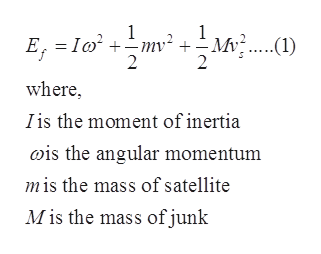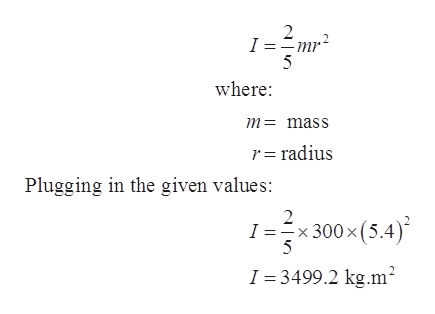# A spherical satellite of approximately uniform density with radius 5.4 m and mass 300 kg is originally moving with velocity <2600, 0, 0>m/s, and is originally rotating with an angular speed 2 rad/s, in the direction shown in the diagram. A small piece of space junk of mass 5.1 kg is initially moving toward the satellite with velocity <−2200, 0, 0>m/s. The space junk hits the edge of the satellite as shown in the figure below, and moves off with a new velocity <−1300, 480, 0>m/s. Both before and after the collision, the rotation of the space junk is negligible.What is the rise in the internal energy of the satellite and space junk combined?

Question
17 views

A spherical satellite of approximately uniform density with radius 5.4 m and mass 300 kg is originally moving with velocity <2600, 0, 0>m/s, and is originally rotating with an angular speed 2 rad/s, in the direction shown in the diagram. A small piece of space junk of mass 5.1 kg is initially moving toward the satellite with velocity <−2200, 0, 0>m/s. The space junk hits the edge of the satellite as shown in the figure below, and moves off with a new velocity <−1300, 480, 0>m/s. Both before and after the collision, the rotation of the space junk is negligible.

What is the rise in the internal energy of the satellite and space junk combined?

check_circle

Step 1

Given data:

Radius of satellite = 5.4 m

Mass of satellite = 300 kg

Velocity = 2600 m/s

Initial angular speed = 2 rad/s

Mass of junk = 5.1 kg

New velocity =  2200

Step 2

Total energy of the system after they combinedhelp_outlineImage Transcriptionclose1 E I mv2 + - Mv ....(1) 2 2 where, I is the moment of inertia is the angular momentum mis the mass of satellite M is the mass of junk fullscreen
Step 3

Moment of inertia of the satelli...help_outlineImage Transcriptionclose2 5 where m= mass r= radius Plugging in the given values: 2 I -x 300 x (5.4) I 3499.2 kg.m fullscreen

### Want to see the full answer?

See Solution

#### Want to see this answer and more?

Solutions are written by subject experts who are available 24/7. Questions are typically answered within 1 hour.*

See Solution
*Response times may vary by subject and question.
Tagged in

### Physics